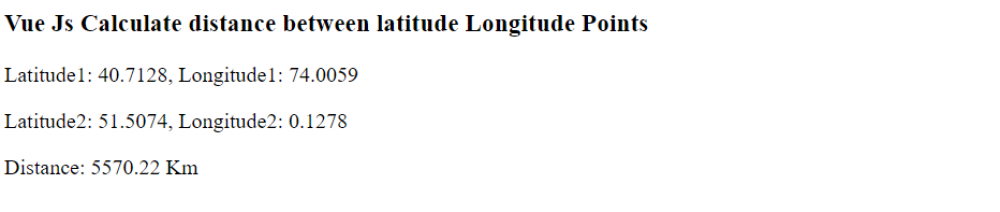# Vue Js Calculate Distance Between Latitude Longitude Points

Vue Js Calculate Distance Between latitude and longitude Points : We can use the Haversine formula to calculate the distance between two points given their latitude and longitude coordinate.

The Haversine formula is a mathematical formula that is used to calculate the great-circle distance between two points on a sphere, such as the Earth. Given the latitude and longitude coordinates of two points, the formula can be used to determine the shortest distance between those two points along the surface of the sphere.

The formula takes into account the Earth's radius, as well as the differences in the latitude and longitude of the two points

## How does the calculation method determine the distance between the two coordinates?

The code you provided is a Vue.js application that calculates the distance between two coordinates on Earth, represented by latitude and longitude values.

The user interface of the application is defined in the HTML code with a Vue template, where it displays the two sets of latitude and longitude values, as well as the calculated distance between them.

The JavaScript code implements the logic for the calculation of the distance. It does so by first converting the latitude and longitude values from degrees to radians, then it applies the Haversine formula to determine the distance between the two points on the Earth's surface. The formula takes into account the Earth's radius and the latitude and longitude values of the two points. The result is then returned in kilometers and rounded to two decimal places.

In simple terms, the code creates a Vue application, sets the latitude and longitude values for two points, then calculates and displays the distance between them in kilometers.

### Vue.js Distance Calculation between Coordinates on Earth

Copied to Clipboard

### Output of above example